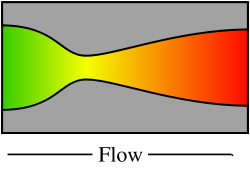## FANDOM

655 PagesDiagram of a de Laval nozzle, showing approximate flow velocity increasing from green to red in the direction of flow

A de Laval nozzle (or convergent-divergent nozzle, CD nozzle or con-di nozzle) is a tube that is pinched in the middle, making an hourglass-shape.

## How usedEdit

It is used as a means of accelerating the flow of a gas passing through it.

## Where usedEdit

It is widely used in some types of steam turbine and is an essential part of the modern rocket engine and supersonic jet engines.

## HistoryEdit

The nozzle was developed by Swedish  inventor Gustaf de Laval  in the 19th century.

## How it worksEdit

Its operation relies on the different properties of gases flowing at subsonic and supersonic speeds. The speed of a subsonic flow of gas will increase if the pipe carrying it narrows because the mass flow rate is constant (grams or pounds per second). The gas flow through a de Laval nozzle is isentropic (gas entropy is nearly constant) and adiabatic (heat loss or gain is nearly zero). At subsonic flow the gas is compressible; sound, a small pressure wave, will propagate through it. Near the nozzle "throat", where the cross sectional area is a minimum, the gas velocity locally becomes transonic (Mach number = 1.0), a condition called choked flow. As the nozzle cross sectional area increases the gas continues to expand and the gas flow increases to supersonic velocities where a sound wave will not propagate backwards through the gas as viewed in the rest frame of the nozzle (Mach number > 1.0).

A de Laval nozzle using hot air at a pressure of 1,000 psi (6.9 MPa or 68 atm), temperature of 1470 K, would have a pressure of 540 psi (3.7 MPa or 37 atm), temperature of 1269 K at the throat, and 15 psi (0.1 MPa or 1 atm), temperature of 502 K at the nozzle exit. The expansion ratio, nozzle cross sectional area at exit divided by area at throat, would be 6.8. The specific impulse would be 151 s (1480 N•s/kg).

This principle was used in a rocket engine by Robert Goddard . Walter Thiel's  implementation of it made the V2 rocket  possible.

## Conditions for operationEdit

A de Laval nozzle will only choke at the throat if the mass flow through the nozzle is sufficient, otherwise no supersonic flow is achieved.

In addition, the pressure of the gas at the exit of the expansion portion of the exhaust of a nozzle must not be too low. Because pressure cannot travel upstream through the supersonic flow, the exit pressure can be significantly below ambient pressure it exhausts into, but if it is too far below ambient, then the flow will cease to be sonic, even within the expansion portion of the nozzle. In practice ambient pressure must be no higher than roughly 2.7 times the pressure in the supersonic gas for supersonic flow to leave the nozzle.

## Analysis of gas flow in de Laval nozzlesEdit

The analysis of gas flow through de Laval nozzles involves a number of concepts and assumptions:

• For simplicity, the combustion gas is assumed to be an ideal gas.
• The gas flow is isentropic (i.e., at constant entropy), frictionless, and adiabatic (i.e., there is little or no heat gained or lost)
• The gas flow is constant (i.e., steady) during the period of the propellent burn.
• The gas flow is along a straight line from gas inlet to exhaust gas exit (i.e., along the nozzle's axis of symmetry)
• The gas flow behavior is compressible since the flow is at very high velocities.

## Exhaust gas velocityEdit

As the combustion gas enters a nozzle, it is traveling at subsonic velocities. As the throat contracts down the gas is forced to accelerate until at the nozzle throat, where the cross-sectional area is the smallest, the linear velocity becomes sonic. From the throat the cross-sectional area then increases, the gas expands and the linear velocity becomes progressively more supersonic.

The linear velocity of the exiting exhaust gases can be calculated using the following equation:

$V_e = \sqrt{\;\frac{T\;R}{M}\cdot\frac{2\;k}{k-1}\cdot\bigg[ 1-(P_e/P)^{(k-1)/k}\bigg]}$
 Ve where: = Exhaust velocity at nozzle exit, m/s = absolute temperature of inlet gas, K = Universal gas law constant = 8314.5 J/(kmol·K) = the gas molecular mass, kg/kmol    (also known as the molecular weight) = cp/cv = isentropic expansion factor = specific heat of the gas at constant pressure = specific heat of the gas at constant volume = absolute pressure of exhaust gas at nozzle exit, Pa = absolute pressure of inlet gas, Pa

Some typical values of the exhaust gas velocity Ve for rocket engines burning various propellants are:

As a note of interest, Ve is sometimes referred to as the ideal exhaust gas velocity because it based on the assumption that the exhaust gas behaves as an ideal gas.

As an example calculation using the above equation, assume that the propellant combustion gases are: at an absolute pressure entering the nozzle of P = 7.0 MPa and exit the rocket exhaust at an absolute pressure of Pe = 0.1 MPa; at an absolute temperature of T = 3500 K; with an isentropic expansion factor of k = 1.22 and a molar mass of M = 22 kg/kmol. Using those values in the above equation yields an exhaust velocity Ve = 3181 m/s or 3.18 km/s which is consistent with above typical values.

The technical literature can be very confusing because many authors fail to explain whether they are using the universal gas law constant R which applies to any ideal gas or whether they are using the gas law constant Rs which only applies to a specific individual gas. The relationship between the two constants is Rs = R/M.

##Edit

Community content is available under CC-BY-SA unless otherwise noted.